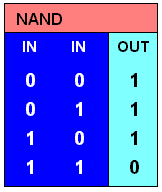# NAND

(redirected from NAND gates)
Also found in: Dictionary.
Related to NAND gates: Logic gates

## NAND

[nand]
(mathematics)
A logic operator having the characteristic that if P, Q, R, … are statements, then the NAND of P, Q, R, … is true if at least one statement is false, false if all statements are true. Derived from NOT-AND. Also known as sheffer stroke.
McGraw-Hill Dictionary of Scientific & Technical Terms, 6E, Copyright © 2003 by The McGraw-Hill Companies, Inc.

## NAND

Not AND.

The Boolean function which is true unless both its arguments are true, the logical complement of AND:

A NAND B = NOT (A AND B) = (NOT A) OR (NOT B)

Its truth table is:

A | B | A NAND B --+---+--------- F | F | T F | T | T T | F | T T | T | F

NAND, like NOR, forms a complete set of Boolean functions on its own since it can be used to make NOT, AND, OR and any other Boolean function:

NOT A = A NAND A

A AND B = NOT (A NAND B)

A OR B = (NOT A) NAND (NOT B)
This article is provided by FOLDOC - Free Online Dictionary of Computing (foldoc.org)

## NAND

(1) See NAND flash.

(2) (Not AND) A Boolean logic operation that is true if any one of its two inputs is false. A NAND gate is constructed of an AND gate followed by a NOT gate. All logic operations can be created from NAND gates. See Boolean logic and NAND flash.Copyright © 1981-2019 by The Computer Language Company Inc. All Rights reserved. THIS DEFINITION IS FOR PERSONAL USE ONLY. All other reproduction is strictly prohibited without permission from the publisher.
References in periodicals archive ?
According to GSW scheme, we obtain a leveled fully homomorphic encryption scheme which is a circuit of depth- L with NAND gates. For the dimension parameter N and the depth parameter L , GSW scheme evaluates depth- Lcircuits of NAND gates with O([N.sup.4+[omega]] * [[kappa].sup.4]) field operations for per gate, where [omega] < 2.3727; In PGSW scheme, the field operations of per gate is O(N), so the time complexity for evaluating depth- L circuits of NAND gates is O(NL) while GSW scheme is O([N.sup.4+[omega]] * [[kappa].sup.4]*L).
Mutant architecture of NAND gates with the four fault models, according to  (a - GOP, b - GOS, c - GOSP, d - GISP)
Regarding the probabilities of each logic gate or sequential component, the average error probability of a NAND gate has been considered to be 0.3314%, while the average failure probability for a D flip-flop has been considered to be 0.1251%.
The fourth step consisted in mutant insertion for each NAND gate, according to the GISP model.
This wires-and-gates stage has two 2-input NAND gates and 2 single wires.
This wire-and-gates stage has two 2-input NAND gates and 1 single wire.
This stage has two 2-input NAND gates. In this stage, PTM is generated by the Kronecker product between PTMs of the NAND gates.
To determine exact error threshold for NAND gate, the automated reliability tool is employed on a series of NAND gates as shown in Figure 8.
In addition, comparing the simulated results of these two categories of CMOS RS flip-flops, CMOS RS flip-flops composed of NAND gates have lower susceptibility to microwave electromagnetic interference than that of CMOS RS flip-flops composed of NOR gates.
An AND gate inverts the signal propagated by a NAND gate while an OR gate inverts that propagated by a NOR gate.
A NAND gate, which stands for "not and," returns a "0" output when all its inputs are "1." It was constructed by the researchers by combining two nanowire switches into a Y-shaped configuration.

Site: Follow: Share:
Open / Close